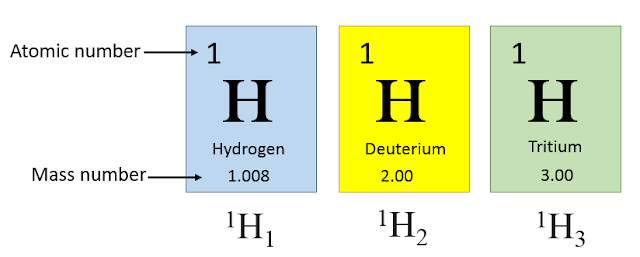# Definition of Mass Number | With Examples | Isoptopes

To better understand the concept of mass number, structure of atom is necessary to learn.

## Definition of mass number

The mass number is the sum of the number of protons and neutrons present in the nucleus of an atom of an element. Each proton and neutron has a mass of 1 amu (atomic mass unit).

The mass number is represented by symbol A, which comes from the German word “Atomgewicht”, where “wicht” means “weight”.

### How to calculate the mass number?

The mass number can easily be calculated by using the following equation:

A = Z + n

Where, A = Mass number, Z = Atomic number, and n = Neutron number.

For example, let's calculate the mass number of hydrogen. Hydrogen has 1 proton and zero neutrons. So the mass number of hydrogen is 1+0 = 1.

Similarly, the mass number of helium is 4 (2+2=4), as it has 2 protons and 2 neutrons in its nucleus.

### Where is the mass number in the periodic table?

The mass number is written below the symbol and name of an element in the periodic table. The mass number of hydrogen and helium are shown in the periodic table are presented in the figure below.Mass numer and atomic number representation of an element in periodic table.

### Examples of mass number

Some examples of the mass number of elements are compiled in the table below.

 Elements Symbols Atomic number (Z) Number of neutrons (n) Mass number (A=Z + n) Hydrogen H 1 0 1 Helium He 2 2 4 Carbon C 6 6 12 Oxygen O 8 8 16 Fluorine F 9 10 19 Sodium Na 11 12 23 Sulphur S 16 16 32

### How to calculate the mass number of isotopes?

Isotopes are the atoms of an element that have the same number of protons but a different number of neutrons in the nucleus. Due to the different number of the neutron, the mass number of isotopes of the same element is different.

### Isotopes of hydrogen

For example, hydrogen has three isotopes i.e., hydrogen, deuterium, and tritium. Hydrogen has zero neutrons, deuterium has 1 neutron, and tritium has 2 neutrons. So the mass number of three isotopes can be calculated as

• Hydrogen = 1 + 0 = 1
• Deuterium = 1 + 1 = 2
• Tritium = 1 + 2 = 3

The mass numbers of three isotopes of hydrogen are shown in the diagram below.Mass numbers of isotopes of hydrogen.